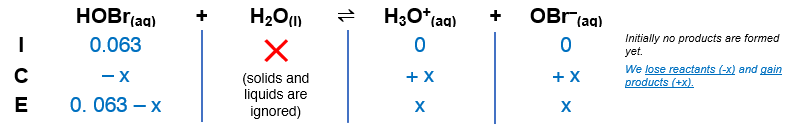# Problem: The pH of a 0.063-M solution of hypobromous acid (HOBr but usually written HBrO) is 4.95. Calculate Ka.

###### FREE Expert Solution

The dissociation of HOBr is as follows:

HOBr(aq) + H2O(l)  H3O+(aq) + OBr(aq)

Step 1. ICE table and Ka expression.The Ka expression for HA is:

$\overline{){{\mathbf{K}}}_{{\mathbf{a}}}{\mathbf{=}}\frac{\mathbf{products}}{\mathbf{reactants}}{\mathbf{=}}\frac{\mathbf{\left[}{\mathbf{H}}_{\mathbf{3}}{\mathbf{O}}^{\mathbf{+}}\mathbf{\right]}\mathbf{\left[}{\mathbf{OBr}}^{\mathbf{-}}\mathbf{\right]}}{\mathbf{\left[}\mathbf{HOBr}\mathbf{\right]}}}$

88% (240 ratings)###### Problem Details

The pH of a 0.063-M solution of hypobromous acid (HOBr but usually written HBrO) is 4.95. Calculate Ka.

Frequently Asked Questions

What scientific concept do you need to know in order to solve this problem?

Our tutors have indicated that to solve this problem you will need to apply the Weak Acids concept. If you need more Weak Acids practice, you can also practice Weak Acids practice problems.

What professor is this problem relevant for?

Based on our data, we think this problem is relevant for Professor Klingler's class at KSU.

What textbook is this problem found in?

Our data indicates that this problem or a close variation was asked in Chemistry: An Atoms First Approach - Zumdahl Atoms 1st 2nd Edition. You can also practice Chemistry: An Atoms First Approach - Zumdahl Atoms 1st 2nd Edition practice problems.